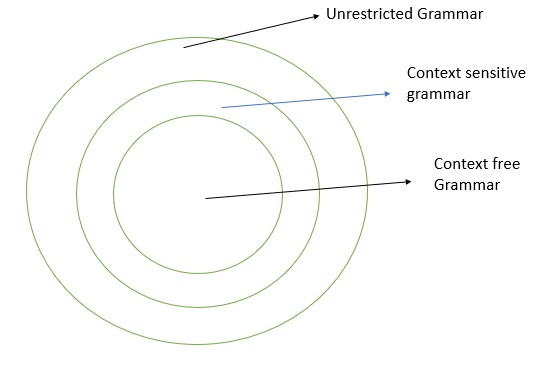# What is Context-sensitive Grammar?

The Context sensitive grammar (CSG) is defined as G=(V,Σ,P,S)

Where,

• V: Non terminals or variables.
• Σ: Input symbols.
• P: Production rule.
• P:{αAβ → αγβ, A ϵ V,α ϵ (V∪Σ)*, β ϵ (V∪Σ)*
• S: Starting symbol.

## Example

• aS→SAa|aA
• aA→abc

In context sensitive grammar, there is either left context or right context (αAβ i.e. α is left context and β is right) with variables.

But in context free grammar (CFG) there will be no context.

For example in production rule

S →0 B S 2 ,

B 0 → 0 B

We cannot replace B until we get B0.

Therefore, CSG is harder to understand than the CFG.

The CFG, CSG and the unrestricted grammar are depicted below −